GMAT Math : Arithmetic

Example Questions

Example Question #61 : Arithmetic

Jerry is a Cardinal fan and he and his family live on a street with 9 other families that are all Cardinal fans. One block to the north, there are 11 families and they are all Cub fans. These 21 households all buy their lawn fertilizer from Ben's Lawn and Garden Shop. Jerry suspects that Ben (who is originally from Chicago) is a Cub fan and that he provides better fertilizer to the Cub fans than to the Cardinal fans, while charging the same price for all.

Last Saturday everyone in town mowed their lawn. At 2:00 AM Sunday morning, Jerry snuck around town and weighed all of the grass clippings for the 21 households in question.

The weights (in lbs) of the grass clippings for the 10 Cardinal homes were:

82, 85, 90, 74, 80, 89, 75, 81, 93, 75

The weights (in lbs) of the grass clippings for the 11 Cub homes were:

90, 87, 93, 75, 88, 96, 90, 82, 95, 97, 78

The Cardinal average was 82.4; the Cub average was 88.27.

At what level is the 5.87 lb difference significant? - Asked another way - what is the p value for the 5.87 lb difference.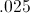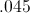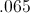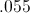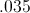Explanation:

Cub variance = 53.218; Cardinal variance = 45.378.

The standard deviation of the difference (5.87) is: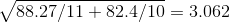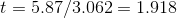TDIST of 1.918 with 19 Degrees of Freedom = .035

So, we would reject the null hypothesis (the hypothesis that claims that the means are equal) at 95% confidence (p=.05) and not reject at 99% (p=.01)

Example Question #62 : Arithmetic

A pair of dice - one fair, one loaded, but each with the usual numbers 1-6 on their faces - are rolled. What is the probability that one die will show a 5 and the other will show a 6?

Statement 1: The probability of rolling a 5 on the loaded die is.

Statement 2: The probability of rolling a 6 on the loaded die is.

EITHER statement ALONE is sufficient to answer the question.

Statement 1 ALONE is sufficient to answer the question, but Statement 2 ALONE is NOT sufficient to answer the question.

BOTH statements TOGETHER are sufficient to answer the question, but NEITHER statement ALONE is sufficient to answer the question.

BOTH statements TOGETHER are insufficient to answer the question.

Statement 2 ALONE is sufficient to answer the question, but Statement 1 ALONE is NOT sufficient to answer the question.

BOTH statements TOGETHER are sufficient to answer the question, but NEITHER statement ALONE is sufficient to answer the question.

Explanation:

The probability of rolling a 5 and the probability of rolling a 6 on the fair die are both.

Let the probabilities of rolling a 5 and a 6 on the loaded die beand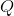, respectively.

The probability of rolling a 6 on the fair die and a 5 on the loaded die is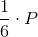The probability of rolling a 5 on the fair die and a 6 on the loaded die is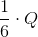Therefore, the probability of rolling a 5-6 one way or the other is their sum: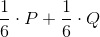which is dependent on both probabilites given in the two statements.

Example Question #63 : Arithmetic

Four cards are removed from a standard deck of fifty-two cards. Did the probability that a randomly drawn card will be a heart increase, decrease, or stay the same?

Statement 1: At least three of the cards are spades.

Statement 2: At least one of the cards is a club.

EITHER statement ALONE is sufficient to answer the question.

BOTH statements TOGETHER are sufficient to answer the question, but NEITHER statement ALONE is sufficient to answer the question.

Statement 1 ALONE is sufficient to answer the question, but Statement 2 ALONE is NOT sufficient to answer the question.

Statement 2 ALONE is sufficient to answer the question, but Statement 1 ALONE is NOT sufficient to answer the question.

BOTH statements TOGETHER are insufficient to answer the question.

BOTH statements TOGETHER are sufficient to answer the question, but NEITHER statement ALONE is sufficient to answer the question.

Explanation:

One-fourth of the cards in a standard deck are hearts; in order to know whether the probability of drawing a heart increased, decreased, or stayed the same, we need to know whether fewer than, exactly, or more than one-fourth of the cards remaining are hearts. Therefore, we need to know whether no hearts, one heart, or more than one heart was removed.

Neither statement alone allows this question to be answered definitively, as we know nothing about the suit(s) of the other card(s). But both statements together tell us that no hearts were removed, so thirteen of the forty-eight cards remaining are hearts. This raises the probability fromto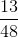.

Example Question #64 : Arithmetic

Four cards are removed from a standard deck of fifty-two cards. Did the probability that a randomly drawn card will be a club increase, decrease, or stay the same?

Statement 1: The four cards that were removed were all kings.

Statement 2: One card of each suit was removed.

Statement 1 ALONE is sufficient to answer the question, but Statement 2 ALONE is NOT sufficient to answer the question.

EITHER statement ALONE is sufficient to answer the question.

Statement 2 ALONE is sufficient to answer the question, but Statement 1 ALONE is NOT sufficient to answer the question.

BOTH statements TOGETHER are insufficient to answer the question.

BOTH statements TOGETHER are sufficient to answer the question, but NEITHER statement ALONE is sufficient to answer the question.

EITHER statement ALONE is sufficient to answer the question.

Explanation:

Thirteen of the fifty-two cards in a standard deck - one-fourth - are clubs, so the probability of drawing a club is.

Either statement tells us that one card of each suit was removed (there is one king of each suit), leaving twelve clubs out of forty-eight cards - one fourth of them. This keeps the probability of drawing a club at.

Example Question #11 : Discrete Probability

A marble is selected at random from a box of red, yellow, and blue marbles. What is the probability that the marble is blue?

Statement 1: There are twice as many red marbles as yellow.

Statement 2: There are three times as many blue marbles as yellow.

BOTH statements TOGETHER are sufficient to answer the question, but NEITHER statement ALONE is sufficient to answer the question.

BOTH statements TOGETHER are insufficient to answer the question.

Statement 1 ALONE is sufficient to answer the question, but Statement 2 ALONE is NOT sufficient to answer the question.

EITHER statement ALONE is sufficient to answer the question.

Statement 2 ALONE is sufficient to answer the question, but Statement 1 ALONE is NOT sufficient to answer the question.

BOTH statements TOGETHER are sufficient to answer the question, but NEITHER statement ALONE is sufficient to answer the question.

Explanation:

The first statement tells us the proportion of red marbles to yellow, and is helpful, but it tells us nothing about the number, relative or absolute, of blue marbles, so it alone does not answer the question; for similar reasons, neither does the second statement alone.

Together, however, they make the picture complete. If there areyellow marbles, then by Statements 1 and 2, there are, respectively,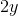red marbles and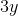blue marbles - and, therefore,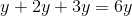marbles total. The probability of drawing a blue marble is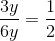.

Example Question #3162 : Gmat Quantitative Reasoning

A marble is selected at random from a box of red, yellow, and blue marbles. What is the probability that the marble is yellow?

Statement 1: Forty of the marbles are not red.

Statement 2: Sixty of the marbles are not blue.

Statement 1 ALONE is sufficient to answer the question, but Statement 2 ALONE is NOT sufficient to answer the question.

BOTH statements TOGETHER are sufficient to answer the question, but NEITHER statement ALONE is sufficient to answer the question.

EITHER statement ALONE is sufficient to answer the question.

BOTH statements TOGETHER are insufficient to answer the question.

Statement 2 ALONE is sufficient to answer the question, but Statement 1 ALONE is NOT sufficient to answer the question.

BOTH statements TOGETHER are insufficient to answer the question.

Explanation:

We need to know the number of marbles that are yellow, and the number of marbles there are total. If we let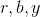be the number of red, blue, and yellow marbles, respectively, the two statements say that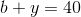and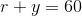These two statements together do not give us enough information. For example, these two situations both fit the conditions given:

Situation 1: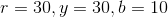, making the probability of drawing a yellow marble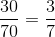Situation 2: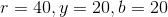, making the probability of drawing a yellow marble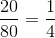Example Question #3163 : Gmat Quantitative Reasoning

One card from another deck is added to a standard deck of fifty-two cards. The cards are shuffled and one card is removed.

A card is then drawn at random. What is the probability that that card is an ace?

Statetment 2: The card that was removed was a jack.

BOTH statements TOGETHER are sufficient to answer the question, but NEITHER statement ALONE is sufficient to answer the question.

Statement 1 ALONE is sufficient to answer the question, but Statement 2 ALONE is NOT sufficient to answer the question.

BOTH statements TOGETHER are insufficient to answer the question.

EITHER statement ALONE is sufficient to answer the question.

Statement 2 ALONE is sufficient to answer the question, but Statement 1 ALONE is NOT sufficient to answer the question.

BOTH statements TOGETHER are insufficient to answer the question.

Explanation:

You need to know two things to answer this question - the rank of the added card, and the rank of the removed card. The second statement is useful but not sufficient; the first is irrelevant to the question.

Example Question #3164 : Gmat Quantitative Reasoning

Two fair dice are rolled. What is the probability that each die (not the sum of the dice) will come up a composite number?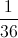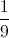Explanation:

There are two composite numbers on each die, 4 and 6. On one die, the probability of rolling a 4 or a 6 is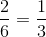; the probabililty of rolling two composite numbers is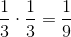.

Example Question #11 : Discrete Probability

Four coins - a fair penny, a loaded penny, a fair nickel, and a loaded nickel - are tossed. What is the probability that all four will come up heads?

Statement 1: Yesterday, out of 100 tosses, the loaded penny came up heads 70 times.

Statement 2: Yesterday, out of 100 tosses, the loaded nickel came up heads 40 times.

EITHER statement ALONE is sufficient to answer the question.

BOTH statements TOGETHER are insufficient to answer the question.

Statement 1 ALONE is sufficient to answer the question, but Statement 2 ALONE is NOT sufficient to answer the question.

BOTH statements TOGETHER are sufficient to answer the question, but NEITHER statement ALONE is sufficient to answer the question.

Statement 2 ALONE is sufficient to answer the question, but Statement 1 ALONE is NOT sufficient to answer the question.

BOTH statements TOGETHER are insufficient to answer the question.

Explanation:

While experiments such as repeated tossings can give an idea of the probability that a coin will come up heads or tails, they do not provide a definitive answer, so neither statement is helpful here.

Example Question #3166 : Gmat Quantitative Reasoning

Two dice are thrown; one is fair, one is loaded, but each has the usual numbers 1-6 on its faces. What is the probability of the outcome being a sum of 2?

Statement 1: The loaded die will come up 1 with probability.

Statement 2: The loaded die will come up any of the numbers 2, 3, 4, 5, or 6 with probability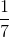each.

BOTH statements TOGETHER are insufficient to answer the question.

Statement 1 ALONE is sufficient to answer the question, but Statement 2 ALONE is NOT sufficient to answer the question.

EITHER statement ALONE is sufficient to answer the question.

Statement 2 ALONE is sufficient to answer the question, but Statement 1 ALONE is NOT sufficient to answer the question.

BOTH statements TOGETHER are sufficient to answer the question, but NEITHER statement ALONE is sufficient to answer the question.

EITHER statement ALONE is sufficient to answer the question.

Explanation:

The two statements are actually equivalent; if the loaded die comes up any of 2, 3, 4, 5, or 6 with probability, then it will come up 1 with probability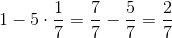, which is exactly the same assertion as Statement 1 makes.

A sum of 2 can only come up one way: a pair of ones. If you know either statement, then you can calculate the probability of tossing a 2 by multiplying, the probability of a die coming up a 1 on the fair die, by, the probability of a 1 coming up on the loaded die.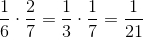Tired of practice problems?

Try live online GMAT prep today.